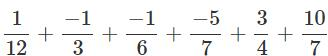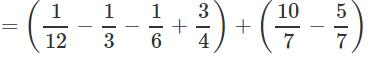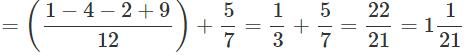Courses

# Test: Rational Numbers- 3

## 20 Questions MCQ Test Mathematics (Maths) Class 8 | Test: Rational Numbers- 3

Description
This mock test of Test: Rational Numbers- 3 for Class 8 helps you for every Class 8 entrance exam. This contains 20 Multiple Choice Questions for Class 8 Test: Rational Numbers- 3 (mcq) to study with solutions a complete question bank. The solved questions answers in this Test: Rational Numbers- 3 quiz give you a good mix of easy questions and tough questions. Class 8 students definitely take this Test: Rational Numbers- 3 exercise for a better result in the exam. You can find other Test: Rational Numbers- 3 extra questions, long questions & short questions for Class 8 on EduRev as well by searching above.
QUESTION: 1

### Which of the following statements is true?

Solution:

Every rational number is not a fraction. In rational numbers, we use integers and in fractions, we use only natural numbers.

QUESTION: 2

### Compare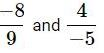Solution:

Expressing in standard form, we get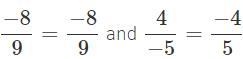Express each rational number with L.C.M as denominator. L.C.M of 9 and 5 is 9 × 5 = 45.

∴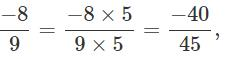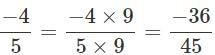Comparing the numerators, we have −40<−36 .

∴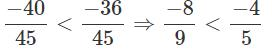QUESTION: 3

### How is -28/84 expressed as a rational number with numerator 4?

Solution:

Given, -28/84
Dividing both the numbers by 7.
Therefore, -4/12.

QUESTION: 4

A Which of the following sets of rational numbers is arranged in ascending order?

Solution:

Convert the given fractions into equivalent fractions and then compare
-25/28 = -25/28
-5/14 = -10/28 (Multiplied both the numerator and denominator by 2)
-3/7 = -12/8 (Multiplied both the numerator and denominator by 4)
-1/2 = -14/28(Multiplied both the numerator and denominator by 14)
Therefore, option (c) is the correct answer.

QUESTION: 5

Which of the following statements is false?

Solution: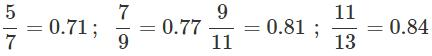So,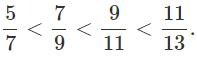Hence, both (b) and (c) are false.

QUESTION: 6

Which of the following is a rational number between 1/4 and 1/3 ?

Solution: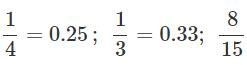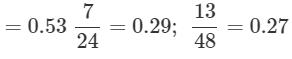QUESTION: 7

Which of the following is the correct arrangement of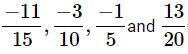in descending order?

Solution:

LCM of 5,10,15 and 20 which is 60. Now making the denominator same, we have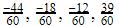. So descending order is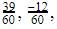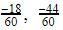that is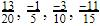QUESTION: 8

Simplify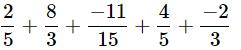Solution:

LCM of 5,3 and 15 is 15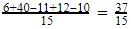QUESTION: 9

What is the sum of the additive inverse and multiplicative inverse of 2?

Solution:

Additive inverse of 2 is −2
Multiplicative inverse of 2 is 1/2
∴ The sum of multiplicative inverse and additive inverse =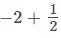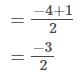Answer :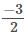QUESTION: 10

Subtract 2/3 from 7/8

Solution:

Solution :- 7/8 - 2/3

= {(21-16)/24}

= 5/24

QUESTION: 11

Which among the following is a rational number equivalent to -5/-3 ?

Solution: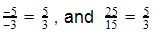Hence the correct answer is 5/3.

QUESTION: 12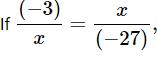what is the Positive value of x?

Solution: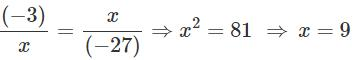QUESTION: 13

Which number is in the middle if -1/6, 4/9, 6/-7, 2/5 and -3/4 arranged in descending order?

Solution:

Solution :- -⅙ = -0.16

4/9 = 0.44

-6/7 = -0.85

⅖ = 0.40

-¾ = -0.75

Descending Order : - 4/9, 2/5, -1/6, -3/4, -6/7

Middle term : - -1/6

QUESTION: 14

What is the average of the two middle rational numbers when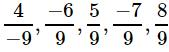and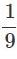are arranged in ascending order?

Solution:

Arranging the given rational numbers in ascending order, we  get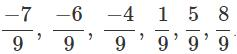The two middle rational numbers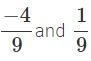Their average is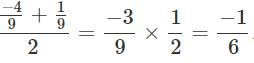QUESTION: 15

Subtract -5/9 from -3/7.

Solution:
QUESTION: 16

What number should be added to -4/7 to get 5/9 ?

Solution:

Let the number be x

Then,

X+(-4/7)=5/9

X=5/9+4/7

X= 35+36/63

X=71/63

QUESTION: 17

What should be subtracted from -7/11 to get −2?

Solution:

Let x be subtracted from -7/11
then -7/11 - x= -2
-x= -2 + (7/11)
-x= -2 + 7/11
-x= -15/11
x= 15/11

QUESTION: 18

By what rational number should -3/5 be multiplied to obtain 21?

Solution:
QUESTION: 19

The sum of two rational numbers is −3. If one of them is -11/5, find the other.

Solution:

Let the other number be x

x+(-11/5)= -3

x= -3+11/5

x=-15+11/5

x= -4/5

QUESTION: 20

Which of the following is the resultant of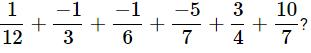Solution: